Home > Blog

# Personalised Week Ahead report from MS Project in Excel

In this blog you will learn how to filter a project or programme plan to show the tasks ahead which have been assignefd to a specific resource.  You will then learn how to colour code this information and distribute it in Excel so that your audience doesn’t need to have MS Project.

## Top-level summary steps

1. Make a filter in Project to display only the tasks next week for a specific resource.
2. Make a view to make running the report simple and repeatable.
3. Make an excel sheet to colour code the report using Conditional Formatting to show tasks starting or finishing next week or those which are late / in play for the whole time
4. Use a Macro to automate the excel process to make running the report very simple and quick.

## What you will need

You will need a project or programme plan which has had resources applied to the tasks in the plan.  The resources assigned to each task need to be all the people that will be involved in the task’s execution (rather than “just” the owners of the task).

In the case of a programme plan with separate inserted (sub) project plans ideally, you would be using a resource pool however this method will work without it.  See this blog for more information about the powerful technique of using a resource pool.

## Making a filter in MS Project

Firstly you need to create a filter in MS Project so that the tasks in the plan can be filtered to show only those that are assigned to your chosen resource.  Obviously, it is useful if you can change the resource which will be used in the filter so you set up the filter to ask who you want to filter on.  This filter will also need to ask you what dates you are interested in.

To set up the filter click on Filter in the View ribbon and chose “New Filter…”.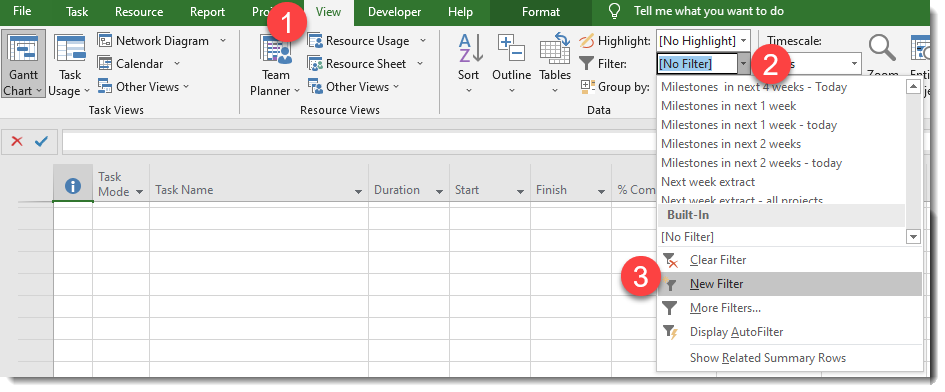This then displays the filter definition dialogue where you can set up the filter.  To cause MS Project to ask you a question you use the format “question you want to ask:”? – note the use of quotes, colon and question mark.  Using this you define a filter that displays any task which fulfils the following criteria:

• AND Will start before date X: is less than or equal to “task starting before:”?
• AND Isn’t complete: is less than 100% complete
• AND Uses resource Y: Resource name contain “Who:”?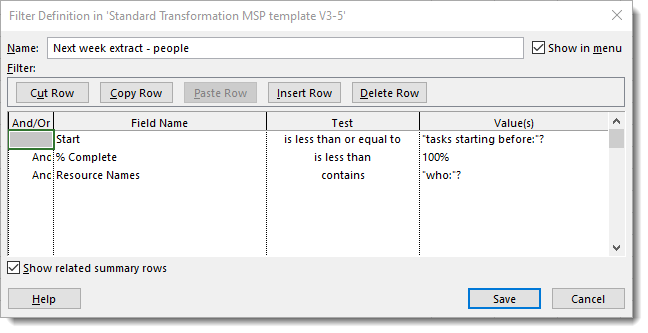Save the filter with a name of your choice, in this case, “Next week extract – people”.

When you have defined the filter you can apply it to the plan.  Note that as you have used “contains” on the name you don’t have to enter the resource’s full name.   If you want to see what is happening next week then simply enter next Friday’s date and all the tasks which start before that date, are not complete and involved the chosen resource will be displayed.

## Make a report in Project

Assuming you are going to be running this filter for multiple people and especially if you are going to be producing it in Excel you will need to produce a View to quickly and easily apply your new filter to the plan and produce consistent output.

To do this you need to set up a new Table and then define a View.

To set up the table click on the Table drop down in the View ribbon and chose “more tables…” and then new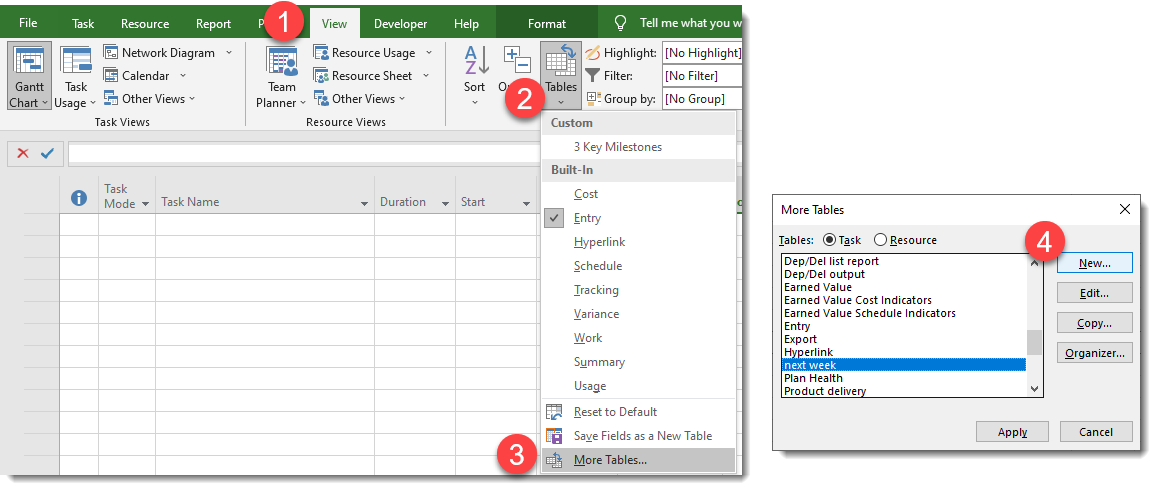Set up a new table displaying the following columns:

ID, Project, Name, Start, Finish, % complete, Resource initials, Summary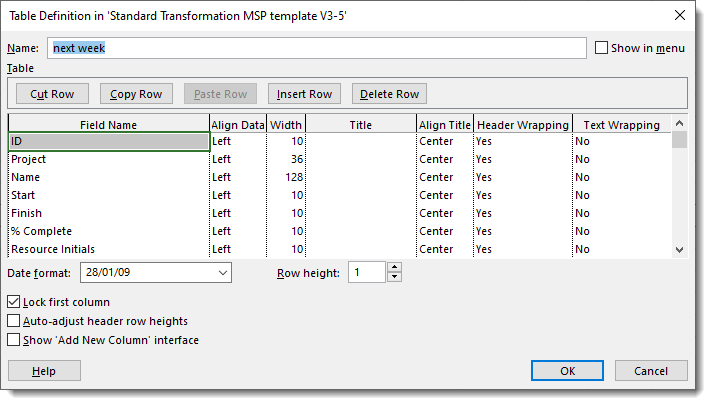If you are going to use the excel option at the end of this blog you need to define the date format to a simple dd/mm/yy format rather than using the default.

Save the table definition with a name of your choice (in this case it is called “next week”.

A View is only a defined collection of Table, Filter and group.  To set up a new View click on the drop-down to on the “Gantt view” button or on the “other views” button and select “more views…” and then New.  You will then be asked if you want a single or combination view; chose “single view”.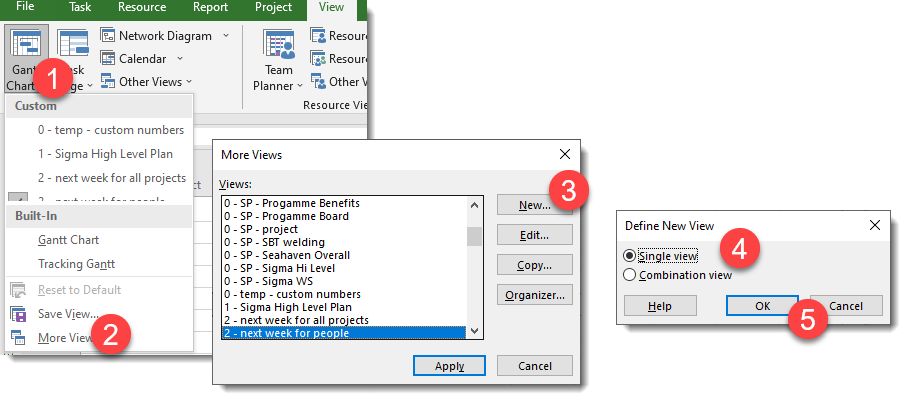Name the view (in this case 2 – next week for people) and then define it by including your new table and filter.  The group is “no group” as we don’t want to apply a grouping and the apply filter as a highlight is left unchecked as we want to hide anything that doesn’t match the filter.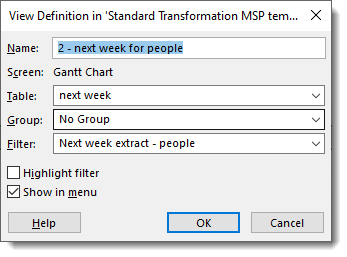Now, whenever you want to see what next week holds for any resource you can click on the view and the table and filter will automatically be applied.

## Colour coding the report in Excel

To produce an attractive report in Excel that can be distributed to people who don’t have MS Project, it is easiest to use Conditional formatting. Copy and paste the output from your new view in MS Project into an Excel sheet.

You need to tell Excel what the date was which was selected, I have put this in cell P2 in this example.

The conditional formatting which needs to be applied to each of the cells are 4 rules to show Late, finishing, starting or in play by the date shown in P2.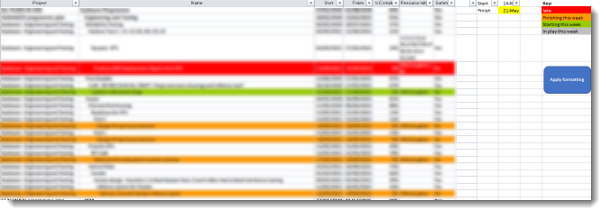The rules for each of these are:

• Red: =AND(\$G1="no",\$D1<\$P\$1,NOT(A1=""),\$E1<100%)
• Amber: =AND(\$G1="no",\$D1<=\$P\$2,NOT(A1=""),\$E1<100%)
• Green: =AND(\$G1="no",\$C1>=\$P\$1,NOT(A1=""),\$E1<100%)
• Grey; =AND(\$G1="no",\$C1<=\$P\$2,NOT(A1=""),\$E1<100%)

All the rules are applied to the whole of the pasted in data, including the first row of titles.

## Automating the paste and formatting

Applying the formatting manually can be a pain, especially as the length of the data will change for each resource.

To speed this up you can use a macro in excel which will

• Delete all the plan data in the sheet
• Paste in the data that you have copied from Excel
• Apply the conditional formatting.

Using a macro means that it is possible to quickly work through a list of resources and save reports.  These can be emailed to them showing what the plan is expecting them to do over the next week.

To input the macro use the keyboard shortcut <ALT><F11> to bring up the VBA window, insert a module in your excel file and paste the code below into the coding pane on the right.   To make the process even faster you can apply a keyboard shortcut to the macro (I use <CTRL><SHIFT><V>) which will trigger the macro.

`Sub clear_paste_format()`

`    Worksheets("Sheet1").Range("b1").Select`

`        'find the last row with a reference`

`    original_lastrow = Range("b65536").End(xlUp).Row`

`   `

`    Range("a2:G" & original_lastrow).ClearContents`

`   `

`    Worksheets("Sheet1").Range("a1").Select`

`    ActiveSheet.Paste`

`       `

`    'Range("a2").PasteSpecial xlPasteValues`

`    'Range("a2").PasteSpecial xlPasteFormats`

`       `

`   `

`    Call apply_conditional_formatting`

`End Sub`

` `

`Sub apply_conditional_formatting()`

`    Worksheets("Sheet1").Range("b1").Select`

`        'find the last row with a reference`

`    lastrow = Range("b65536").End(xlUp).Row`

`    ' Delete all conditional formatting rules in sheet`

`    Cells.FormatConditions.Delete`

`   `

`    'highlight late`

`        With Range("a1:G" & lastrow).FormatConditions.Add(Type:=xlExpression, Formula1:= _`

`          "=AND(\$G1=""no"",\$D1<\$P\$1,NOT(A1=""""),\$E1<100%)")`

`            .Font.Bold = False`

`            .Font.ColorIndex = 2`

`            .Interior.ColorIndex = 3`

`            .StopIfTrue = True`

`           `

`            '"=AND(\$G1=""no"",\$D1<\$P\$1,NOT(A1=""""),\$E1<100%)")`

`        End With`

`    'highlight finish this week`

`        With Range("a1:G" & lastrow).FormatConditions.Add(Type:=xlExpression, Formula1:= _`

`            "=AND(\$G1=""no"",\$D1<=\$P\$2,NOT(A1=""""),\$E1<100%)")`

`            .Font.Bold = False`

`            .Interior.ColorIndex = 45`

`            .StopIfTrue = True`

`        End With`

`        '=AND(\$G1="no",\$D1<=\$P\$2,NOT(A1=""),\$E1<100%)`

`        '"=AND(\$G1=""no"",\$D1<=\$P\$2,NOT(A1=""""),\$E1<100%)")`

`    'highlight start this week`

`        With Range("a1:G" & lastrow).FormatConditions.Add(Type:=xlExpression, Formula1:= _`

`            "=AND(\$G1=""no"",\$C1>=\$P\$1,NOT(A1=""""),\$E1<100%)")`

`            .Font.Bold = False`

`            .Interior.ColorIndex = 43`

`            .StopIfTrue = True`

`        End With`

`   `

`        '=AND(\$G1="no",\$C1>=\$P\$1,NOT(A1=""),\$E1<100%)`

`        '"=AND(\$G1=""no"",\$C1>=\$P\$1,NOT(A1=""""),\$E1<100%)")`

`   `

`    'highlight in play this week`

`        With Range("a1:G" & lastrow).FormatConditions.Add(Type:=xlExpression, Formula1:= _`

`            "=AND(\$G1=""no"",\$C1<=\$P\$2,NOT(A1=""""),\$E1<100%)")`

`            .Font.Bold = False`

`            .Interior.ColorIndex = 15`

`            .StopIfTrue = True`

`        End With`

`        '=AND(\$G1="no",\$C1<=\$P\$2,NOT(A1=""),\$E1<100%)`

`        '"=AND(\$G1=""no"",\$C1<=\$P\$2,NOT(A1=""""),\$E1<100%)")`

`       `

`    'colour Key`

`    Range("R2").Font.ColorIndex = 2`

`    Range("r2").Interior.ColorIndex = 3`

`    Range("r3").Interior.ColorIndex = 45`

`    Range("r4").Interior.ColorIndex = 43`

`    Range("r5").Interior.ColorIndex = 15`

`    ' key entries`

`    Range("R2").Value = "late"`

`    Range("r3").Value = "Finishing this week"`

`    Range("r4").Value = "Starting this week"`

`    Range("r5").Value = "In play this week"`

`   `

`    Columns("A:A").ColumnWidth = 100`

`    Columns("A:A").EntireColumn.AutoFit`

`    With Range("a1:G" & lastrow)`

`        .WrapText = True`

`        .EntireRow.AutoFit`

`    End With`

`End Sub`Miles Goodchild has been a Program and Programme Planner, PMO lead and Project Manger since 1998.  He enjoys using MS Office, especially Project, Excel and Visio to make life easier and simpler.  In the course of this he created SummaryPro.  In this blog he shares some of the tips and techniques he has learnt over the years in the hope that they will be useful to you.